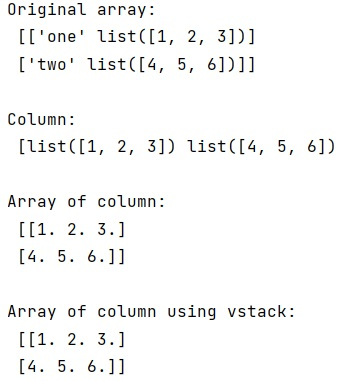# How to convert a NumPy 2D array with object dtype to a regular 2D array of floats?

Learn, how can we convert a given NumPy 2D array with object dtype to a regular 2D array of floats in Python? By Pranit Sharma Last updated : April 04, 2023

Suppose that we are given a numpy ndarray whose each row has a format of 'str' -> ['float']. We need to extract all the float lists and convert them into a single array of data type float. This can be done by using indexing the array and extracting the column containing float lists but the data type would still be Object.

## NumPy 2D array (dtype) to a regular 2D array (floats)

To convert a NumPy 2D array with object dtype to a regular 2D array of floats, we use two approaches, first we can convert the extracted column into a list, so that we can define the data type for this list as float to convert it into a numpy array. Secondly, we can directly use vstack() method on the extracted column which will stack all the lists vertically and we can define the data type as a float without any error.

Let us understand with the help of an example,

## Python code to convert a NumPy 2D array with object dtype to a regular 2D array of floats

```# Import numpy
import numpy as np

# Creating an array
arr = np.array([['one', [1, 2, 3]],['two', [4, 5, 6]]], dtype='O')

# Display array
print("Original array:\n",arr,"\n")

# Extracting column
col = arr[:,1]

# Display column
print("Column:\n",col,"\n")

# Converting col in to array by casting it into a list
res = np.array(list(col),dtype=np.float_)

# Display result
print("Array of column:\n",res,"\n")

# Converting col in to array by using vstack
res = np.vstack(col).astype('float')

# Display result
print("Array of column using vstack:\n",res,"\n")
```

### Output